## Info

Publication number
JP6226366B2
JP6226366B2 JP2013189710A JP2013189710A JP6226366B2 JP 6226366 B2 JP6226366 B2 JP 6226366B2 JP 2013189710 A JP2013189710 A JP 2013189710A JP 2013189710 A JP2013189710 A JP 2013189710A JP 6226366 B2 JP6226366 B2 JP 6226366B2
Authority
JP
Japan
Prior art keywords
reactive power
power
consumer
harmonic
Prior art date
Legal status (The legal status is an assumption and is not a legal conclusion. Google has not performed a legal analysis and makes no representation as to the accuracy of the status listed.)
Active
Application number
JP2013189710A
Other languages
Japanese (ja)
Other versions
JP2015056985A (en
Inventor

Original Assignee

Priority date (The priority date is an assumption and is not a legal conclusion. Google has not performed a legal analysis and makes no representation as to the accuracy of the date listed.)
Filing date
Publication date
Application filed by 株式会社東芝, 東芝インフラシステムズ株式会社 filed Critical 株式会社東芝
Priority to JP2013189710A priority Critical patent/JP6226366B2/en
Publication of JP2015056985A publication Critical patent/JP2015056985A/en
Application granted granted Critical
Publication of JP6226366B2 publication Critical patent/JP6226366B2/en
Active legal-status Critical Current
Anticipated expiration legal-status Critical

• 238000004364 calculation method Methods 0.000 claims description 22
• 238000004458 analytical method Methods 0.000 claims description 9
• 238000009795 derivation Methods 0.000 claims description 6
• 230000014509 gene expression Effects 0.000 description 85
• 238000005259 measurement Methods 0.000 description 55
• 239000011159 matrix material Substances 0.000 description 28
• 238000010586 diagram Methods 0.000 description 16
• 238000004891 communication Methods 0.000 description 7
• 230000005540 biological transmission Effects 0.000 description 5
• 238000000034 method Methods 0.000 description 5
• 230000002093 peripheral Effects 0.000 description 4
• 235000021171 collation Nutrition 0.000 description 2
• 230000004069 differentiation Effects 0.000 description 2
• 238000005070 sampling Methods 0.000 description 2
• 240000001439 Opuntia Species 0.000 description 1
• 238000005516 engineering process Methods 0.000 description 1
• 238000007429 general method Methods 0.000 description 1
• 238000005286 illumination Methods 0.000 description 1
• 239000004973 liquid crystal related substance Substances 0.000 description 1
• 230000004048 modification Effects 0.000 description 1
• 238000006011 modification reaction Methods 0.000 description 1
• 238000004321 preservation Methods 0.000 description 1

## Images

•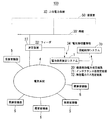•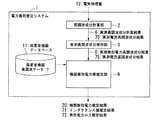•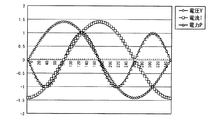•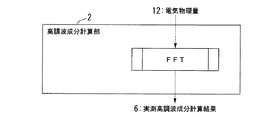•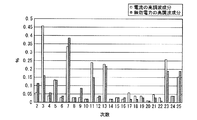•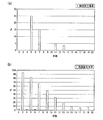•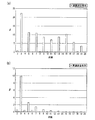•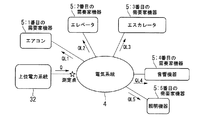•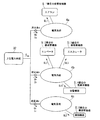•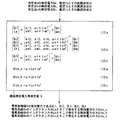•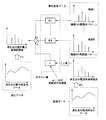•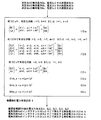•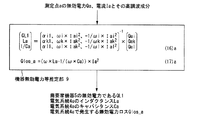•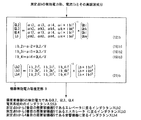•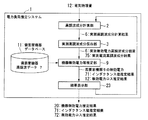•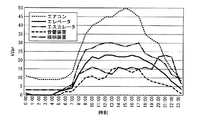•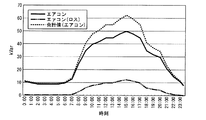•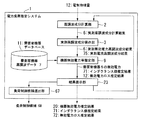•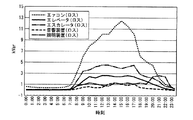•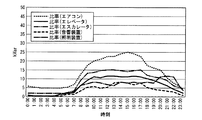## Description

Embodiments described herein relate generally to a power load estimation apparatus.
Areas managed by customers such as buildings with EMS (Energy Management System) such as BEMS (Building and Energy Management System) linked to commercial grid (power company grid) In other words, there is a technique for performing peak cut, peak shift, etc. as power supply and demand control. In this technology, it is necessary to estimate a load amount for each load device of a consumer in order to realize appropriate power supply and demand control.
Japanese Patent No. 4802129 JP 2006-17456 A Japanese Patent No. 3877269
However, when there are many consumer devices or in a wide area, etc., it is often difficult to monitor because a large number of measurement devices and measurement transmission systems are required. For this reason, the system which monitors the load amount of consumer equipment with few measurement points is desired. In addition, when load control such as peak cut or peak shift is performed as supply and demand control, it is necessary to estimate reactive power loss due to reactive power loss of consumer equipment and inductance of distribution lines. is there.
The problem to be solved by the present invention is to provide a power load estimation device that can more easily estimate reactive power of consumer equipment and reactive power loss of distribution lines.
The power load estimation device according to the embodiment is a power load estimation device that estimates a power load amount that is a power load of an electrical system based on a physical quantity measured in an electrical system to which a load device is connected. Unit, a second derivation unit, a data holding unit, and a first estimation unit. The first deriving unit derives a harmonic component of the reactive power from the waveform analysis of the physical quantity of the electric system. The second derivation unit derives a harmonic component of the current from the physical quantity waveform analysis. The data holding unit holds collation data representing the order data of the harmonic components of the reactive power in various load devices. A 1st estimation part estimates the electric power load amount of an electric system by collating the derivation | leading-out result of the harmonic component of a reactive power, and the harmonic component of an electric current with the data for collation.
The block diagram which shows the example of application of the electric power load estimation system of embodiment. The block diagram which shows the structural example of an electric power supply system. The graph which shows the waveform example of an electrophysical quantity. The block diagram which shows the structure of a harmonic component calculation part. The graph which shows the example of a measured reactive power harmonic component result and a measured current harmonic component result. The graph which shows the example of reactive power harmonic data. The graph which shows the example of reactive power harmonic data. The figure which shows the structural example by which consumer equipment is connected to the electrical system. The figure which shows the structural example by which the consumer apparatus is connected to the some electric system. The figure which shows the input-output relationship of estimation parts, such as an apparatus reactive power. Explanatory drawing which shows the outline of the function of the electric power load estimation system containing an estimation part, such as apparatus reactive power. The figure which shows the input-output relationship of estimation parts, such as an apparatus reactive power. The figure which shows the input-output relationship of estimation parts, such as an apparatus reactive power. The figure which shows the input-output relationship of estimation parts, such as an apparatus reactive power. The block diagram which shows another aspect of an electric power load estimation system. The graph of the reactive power for every consumer apparatus displayed on a result display part. The figure which shows the example of a display of the reactive power and reactive power loss for every consumer apparatus which are displayed on a result display part, and those total values. Schematic which shows a structure and function of an electric power load estimation system. The figure of the example which displayed the ratio of the sum of the reactive power and the reactive power loss with respect to the power consumption of the apparatus in a consumer. The figure of the example which displayed the ratio of the sum of the reactive power with respect to the power consumption of the apparatus in a consumer, and a reactive power loss.
(1. First embodiment)
Hereinafter, a power load estimation system according to an embodiment will be described with reference to the drawings.
FIG. 1 is a block diagram showing a configuration of the power supply system 100.
In the power supply system 100, the power load estimation system 1 is connected to a consumer's electrical system 4 that is electrically connected to the feeder 22 of the consumer 50 that uses power. The power load estimation system 1 receives power from, for example, a higher power system 32 made of a commercial system, and estimates the load amount of the customer's electric system 4. The consumer's electrical system 4 is an electrical system provided in a building of a consumer 50 or a general house.
The power load estimation system 1 can be configured by a computer on which a program is installed, for example. The power load estimation system 1 includes a program for estimating reactive power supplied to the consumer device 5 and a program for estimating an inductance value and reactive power loss between the consumer device 5 and the measuring device 27. Yes.
The measuring device 27 measures the electrical physical quantity 12 such as current, voltage, and power from the feeder 22 electrically connected to the bus bar 30 of the customer 50, and uses the information related to the measured electrical physical quantity 12 as the electrical physical quantity information 34 as a power load. It transmits to the estimation system 1.
The power load estimation system 1 estimates the reactive power supplied to the consumer device 5 based on the electrical physical quantity information 34 received from the measurement device 27, the inductance value between the consumer device 5 and the measurement device 27, and Estimate reactive power loss.
The power load estimation system 1 transmits the estimated reactive power supplied to the consumer device 5 to the supply and demand control system 70 as the device reactive power estimation result 20. The power load estimation system 1 transmits the estimated inductance value between the consumer device 5 and the measuring device 27 to the supply and demand control system 70 as the inductance value estimation result 71. The power load estimation system 1 transmits the estimated reactive power loss between the consumer device 5 and the measuring device 27 to the supply and demand control system 70 as the reactive power loss estimation result 72.
The supply and demand control system 70 performs power supply and demand control such as peak cut and peak shift based on the received device reactive power estimation result 20, the inductance value estimation result 71, and the reactive power loss estimation result 72.
FIG. 2 is a block diagram illustrating a configuration example of the power load estimation system 1.
The power load estimation system 1 includes a harmonic component calculation unit 2, an actually measured harmonic component storage unit 3, a consumer device reactive power estimation unit 9, and a customer device database 11.
The harmonic component calculation unit 2 executes a waveform analysis process based on the electrical physical quantity 12 measured from the customer's electrical system 4, and generates a reactive power harmonic component (measured reactive power harmonic component result 6) or current A harmonic component (measured current harmonic component result 75) is calculated. The harmonic component calculation unit 2 outputs the measured reactive power harmonic component result 6 and the measured current harmonic component result 75 to the measured harmonic component storage unit 3.
The measured harmonic component storage unit 3 stores the measured reactive power harmonic component result 6 and the measured current harmonic component result 75 output from the harmonic component calculation unit 2.
The device reactive power etc. estimation unit 9 includes the measured reactive power harmonic component result 6 and the measured current harmonic component result 75 stored in the measured harmonic component storage unit 3, and the reactive power harmonic stored in the consumer device database 11. Based on the wave data 7, the device reactive power estimation result 20, the inductance value estimation result 71, and the reactive power loss estimation result 72 are estimated, and the device reactive power etc. estimation result 20, the inductance value estimation result 71, and the reactive power are estimated. The loss estimation result 72 is output. The customer equipment database 11 will be described later.
FIG. 3 is a graph showing a waveform example of the electrical physical quantity 12. In FIG. 3, waveform examples of the voltage V, current I, and power P are shown as an example of the electrical physical quantity 12. In FIG. 3, the horizontal axis indicates time, and the vertical axis indicates the magnitude of the electrical physical quantity 12.
When instantaneous values of voltage V, current I, and power P are v, i, and p, respectively, the following equation (1) is established.
Based on the equation (1), the instantaneous value p of power can be calculated from the instantaneous value v of voltage and the instantaneous value i of current in the physical quantity 12.
FIG. 4 is a block diagram illustrating a configuration of the harmonic component calculation unit 2 of the power load estimation system 1. The harmonic component calculation unit 2 executes a fast Fourier transform FFT (Fast Fourier Transform) based on the electric physical quantity 12 measured and inputted in the customer's electric system 4, and the order of the harmonics included in the electric physical quantity 12. Calculate each component. For any of v, i, and p as the electrophysical quantity 12, it is possible to calculate components for each harmonic order. Note that the method of calculating components for each harmonic order is not limited to the fast Fourier transform FFT.
The fast Fourier transform FFT will be described. By the fast Fourier transform FFT, the harmonics are represented as a collection of sine waves having different periods. If the fundamental wave is primary, the period is 1/2 of the fundamental wave (frequency is twice), the period is 1/3 of the fundamental wave (frequency is 3 times), the third order, 1 / n (frequency is n Times) is called the nth order.
When a harmonic component enters the commercial frequency (for example, 50 Hz) of the power system, the commercial frequency is called distorted. The harmonic component entering the commercial frequency of the power system can be calculated by Fourier transform. When the observation data is a sampling value, when a harmonic component is determined from the sampling value, a technique called discrete Fourier transform is used. A technique improved so that the discrete Fourier transform can be solved at high speed is called a fast Fourier transform FFT, which is the most versatile technique in harmonic analysis.
Harmonic components in the customer's electrical system 4 are generated and mixed by the consumer device 5 (various electrical devices), but depending on the type of the consumer device 5, the phase of voltage and current during operation differs. . In the consumer device 5 having a large phase difference between the voltage and current during operation, a harmonic component of the reactive power Q appears as in the following equation (1) ′.
FIG. 5 is a graph showing an example of the measured reactive power harmonic component result 6 and the measured current harmonic component result 75 calculated by the harmonic component calculation unit 2. FIG. 5 calculates the component for each harmonic order based on the electrophysical quantity 12 shown in FIG. 3, and the intensity of the harmonic of each order is the total sum of the harmonics including the fundamental wave. It is converted into a harmonic content indicating whether the ratio is indicated. The harmonic content can be expressed by the following equation (2).
In FIG. 5, the vertical axis represents the harmonic content and the horizontal axis represents the harmonic order. In the example of FIG. 5, the harmonic content of reactive power Q and the harmonic content of current I are shown.
The consumer equipment database 11 of the power load estimation system 1 will be described. The consumer equipment database 11 stores reactive power harmonic data 7 indicating the harmonic content of reactive power of the consumer equipment 5.
FIG.6 and FIG.7 is a graph which shows the example of the reactive power harmonic data 7 which shows the harmonic content rate of reactive power about the different consumer apparatus 5, respectively. 6A is an example of an acoustic device, FIG. 6B is an example of an elevator, FIG. 7A is an example of an air conditioner (hereinafter abbreviated as “air conditioner”), and FIG. 7B is an illumination. It is an example of an apparatus. In any graph, the vertical axis represents the harmonic content, and the horizontal axis represents the harmonic order.
The device reactive power estimation unit 9 of the power load estimation system 1 will be described.
If the harmonic content of the i-th order calculated from the equation (2) is αi, the reactive power of the consumer device 5 is QL, and the magnitude of the harmonic of the i-th order is QL_i, There is a relationship of the following formula (3) among αi, QL, and QL_i.
Assuming that the Nth-order harmonic is included in the reactive power of the consumer device 5, the relationship of the following equation (4) can be shown from the equation (3).
Further, if the reactive power in the j-th consumer device 5 is QL j , the i-th reactive power in the j-th consumer device 5 is QL ji, and the harmonic content of the i-th order is α ij. There is a relationship of the following formula (5).
FIG. 8 is a diagram illustrating a configuration example in which five consumer devices 5 are connected to the electrical system 4 in the consumer. In FIG. 8, it is assumed that power is supplied from the upper power system 32 via the electrical system 4 to the first to fifth consumer devices 5 existing in the consumer.
As shown in FIG. 8, the reactive power supplied to the air conditioner which is the first consumer device 5 is QL1, the reactive power supplied to the elevator which is the second consumer device 5 is QL2, and the third demand. The reactive power supplied to the escalator which is the home device 5 is supplied to QL3, the reactive power supplied to the acoustic device which is the fourth consumer device 5 is supplied to the lighting device which is QL4 and the fifth consumer device 5. The reactive power is QL5.
FIG. 9 is a diagram illustrating a configuration example in which five consumer devices 5 are connected to a plurality of electrical systems 4a to 4c in the consumer. In FIG. 9, it is assumed that power is supplied from the upper power system 32 to the first to fifth consumer devices 5 existing in the consumer via the electrical systems 4a to 4c.
The plurality of electric systems 4a to 4c are, for example, an electric system for an air conditioner (electric system 4a) for connecting an air conditioner, an electric system (electric system 4b) for connecting an escalator, etc. Examples include dividing the electrical system 4c).
As shown in FIG. 9, the air conditioner that is the first consumer device 5 is supplied with reactive power QL1 through the measurement system a and the electrical system 4a. The reactive power passing through the measurement point a is Qa, and the current passing through the measurement point a is Ia.
Reactive power QL2 is supplied to the elevator which is the second consumer device 5 through the measurement point b and the electric system 4b. The escalator which is the third consumer device 5 is supplied with reactive power QL3 via the electric system 4b through the measurement point b. The reactive power QL4 is supplied to the acoustic device which is the fourth consumer device 5 through the measurement point b and the electric system 4b. The reactive power passing through the measurement point b is Qb and the current is Ib.
The reactive power QL5 is supplied to the lighting device which is the fifth consumer device 5 through the measurement system c and the electric system 4c. The reactive power passing through the measurement point c is Qc, and the current passing through the measurement point c is Ic.
The reactive power supplied to the air conditioner that is the first consumer device 5 is defined as QL1, and the reactive power loss that occurs when the reactive power QL1 passes through the electrical system 4a between the reactive power Qa that passes through the measurement point a. Assuming Qloss1, there is a relationship of the following formula (6) a.
The reactive power supplied to the elevator which is the second consumer device 5 is QL2, and the reactive power supplied to the escalator which is the third consumer device 5 is QL3. The reactive power supplied to a certain audio equipment is assumed to be QL4.
The reactive power loss generated when the reactive power QL2 passes through the electric system 4b with respect to the reactive power Qb passing through the measurement point b is defined as Qlos2, and the reactive power loss generated when the reactive power QL3 passes through the electric system 4b is defined as Qloss3. Assuming that the reactive power loss generated when the reactive power QL4 passes through the electric system 4b is Qlos4, the relationship of the above equation (6) b is established.
Reactive power generated by the reactive power QL5 passing through the electric system 4c between the reactive power QL5 supplied to the lighting equipment as the fifth consumer equipment 5 and the reactive power Qc passing through the measurement point c When the loss is Qloss5, there is a relationship of the above formula (6) c.
Next, Formula (6) a, Formula (6) b, and Formula (6) c are developed for each harmonic order.
Assuming that the i-th harmonic of Qa is Qai, the i-th harmonic of QL1 is QLi1, and the Q-th harmonic of Qlos1 is Qlossi1, the equation (6) a is expressed by the following equation (7) a.
Similarly, Expression (6) b becomes Expression (7) b, and Expression (6) c becomes Expression (7) b.
Assuming that the inductance from the measurement point a to the air conditioner as the first consumer device 5 through the electrical system 4a is La and the i-th harmonic of Ia is Iai, Equation (7) a is expressed by the following equation ( 8) a ω i is the angular velocity of the i th harmonic.
From the measurement point b to the elevator that is the second consumer device 5, the escalator that is the third consumer device 5, and the acoustic device that is the fourth consumer device 5 through the electric system 4b If the inductance of Lb is Lb and the i-th harmonic of Ib is Ibi, Equation (7) b becomes Equation (8) b.
Further, assuming that the inductance from the measurement point c through the electrical system 4c to the lighting device which is the fifth consumer device 5 is Lc, and the i-th harmonic of Ic is Ici, Equation (7) b is (8) Expression c.
Therefore, the following formula (9) a, formula (9) b, and formula (9) c are derived from these.
In Expression (9) a, what we want to find is two values of QL1 and La, and in Expression (9) b, we want to find four values of QL2, QL3, QL4 and Lb. ) What we want to find in c is the two values QL5 and Lc.
Therefore, to obtain two values of QL1 and La using equation (9) a, an equation consisting of two or more equations, equation (9) b, is used to obtain four values of QL2, QL3, QL4, and Lb. In order to obtain one value, an equation consisting of four or more equations, equation (9) c, and using two equations QL5 and Lc, an equation consisting of two or more equations is required.
Using Equation (9) a, two values of QL1 and La are obtained.
Further, when Expression (9) a is expressed by i-order harmonic and k-order harmonic, Expression (9) a becomes Expression (10) a1 and Expression (10) a2, respectively.
When Expression (9) b is expressed by harmonics of i-order, k-order, l-order, and m-order, Expression (9) b is expressed by Expression (10) b1, Expression (10) b2, Expression (10) b3, respectively. (10) b4.
When Expression (9) c is expressed by the i-order harmonic and the k-order harmonic, Expression (9) c becomes Expression (10) c1 and Expression (10) c2, respectively.
Expressions (10) a1 and (10) a2 are simultaneous equations of variable 2, and the solution is obtained because there are two independent expressions. Further, equation (10) b1, equation (10) b2, equation (10) b3, equation (10) b4 are simultaneous equations of variable 4, and a solution is obtained because there are four independent equations. Expressions (10) c1 and (10) c2 are also simultaneous equations of variable 2, and a solution can be obtained because there are two independent expressions.
If Expression (10) a1 and Expression (10) a2 are expressed as a matrix, the following Expression (11) a is obtained, and Expression (10) b1, Expression (10) b2, Expression (10) b3, and Expression (10) b4 are expressed as follows. When expressed in a matrix, the following expression (11) b is obtained, and when expression (10) c1 and expression (10) c2 are expressed in a matrix, the following expression (11) c is obtained.
Formula (10) a1-Formula (10) a2, Formula (10) b1- Formula (10) b4, and Formula (10) c1- Formula (10) c2 are independent formulas. Therefore, each column of Expression (11) a to Expression (11) c is a regular matrix having an inverse matrix. Therefore, the following formulas (12) a to (12) c are obtained.
By solving the equations (12) a to (12) c, QL1 and La are obtained from the equation (12) a, QL2, QL3, QL4, and Lb are obtained from the equation (12) b, and from the equation (12) c. QL5 and Lc can be obtained.
Since the current Ia passing through the measurement point a in FIG. 9, the current Ib passing through the measurement point b, and the current Ic passing through the measurement point c are known, the first consumer equipment 5 passes through the electrical system 4a from the measurement point a. The loss of reactive power reaching the air conditioner is Qloss_a, passing through the electrical system 4b from the measurement point b, the elevator as the second consumer device 5, the escalator as the third consumer device 5, and the fourth demand Assuming that the reactive power loss reaching the acoustic device which is the home device 5 is Qlos_b, and the reactive power loss reaching the lighting device which is the fifth consumer device 5 from the measurement point c through the electric system 4c is Qlos_c, Qloss_a, Qlos_b, Qlos_c can be obtained from the following equation (13) a, equation (13) b, and equation (13) c, respectively.
FIG. 10 is a diagram illustrating the input / output relationship of the device reactive power estimation unit 9. The input to the device reactive power estimation unit 9 includes the reactive power Qa at the measurement point a, its harmonic component and current Ia and its harmonic component, the reactive power Qb at the measurement point b, its harmonic component, current Ib and its harmonic. These are the wave component, reactive power Qc at the measurement point c, its harmonic component, current Ic, and its harmonic component. On the other hand, the output of the device reactive power estimation unit 9 includes QL1, QL2, QL3, QL4, and QL5 which are reactive power of the consumer device 5, an inductance La of the electric system 4a, and a reactive power loss generated there Qloss_a, the inductance Lb of the electric system 4b and the reactive power loss Qlos_b generated there, and the inductance Lc of the electric system 4c and the reactive power loss Qlos_c generated there. The device reactive power estimation unit 9 obtains each output value by solving the equations (12) a to (12) c and (13) a to (13) c.
The device reactive power etc. estimation unit 9 is the reactive power of the consumer device 5, QL1, QL2, QL3, QL4, QL5, inductance La of the electric system 4a, reactive power loss Qlos_a generated there, and inductance of the electric system 4b Lb and the reactive power loss Qlos_b generated therein, the inductance Lc of the electric system 4c, the reactive power loss generated there, and Qlos_c are output as the estimation result 20 of the device reactive power etc.
FIG. 11 is an explanatory diagram illustrating an outline of functions of the power load estimation system 1 including the device reactive power estimation unit 9.
The total value obtained by multiplying the harmonic pattern of each device (device 1 to device N) existing in the customer device database 11 by the reactive power of the customer device 5 and the square of the harmonic of the current at the measurement point The reactive power of the consumer device 5 and the inductance L of the electrical system are obtained so that the sum of the products of the inductances L becomes a harmonic of the reactive power at the measurement point.
For the power at the measurement point shown in FIG. 11, the waveform of the power P shown in FIG. 3, the reactive power actual harmonic at the measurement point is the harmonic component of the reactive power (FIG. 5), and the current harmonic at the measurement point is the current harmonic. The harmonic pattern (refer FIGS. 6-7) of the apparatus 1-the apparatus N used as a wave component (FIG. 5) is used. The harmonic patterns of the devices 1 to N are represented by the matrix [α ij ] in the equations (12) a to (12) c. As long as such a harmonic pattern is acquired in advance, the creation method is arbitrary.
6-7, the consumer equipment harmonic component 7 of the consumer equipment 5 which changes for every equipment was shown. The harmonic pattern shown in this graph has characteristics for each consumer device 5.
For example, the acoustic device in FIG. 6A is characterized by the magnitudes of components of orders 5, 7, 11, and 13. The elevator shown in FIG. 6B is characterized by the magnitudes of components of orders 3, 5, 7, 9, 11, 13, 15, 17, and 19. The air conditioner of FIG. 7A is characterized by the component sizes of orders 3, 5, 7, 9, 11, 13, 15, 17, and 19. The lighting apparatus of FIG. 7B is characterized by the size of components of orders 3, 5, 7, 9, and 11.
Thus, if the order with a large component is selected and calculated, the reactive power of the consumer device 5 can be easily obtained. For example, the acoustic equipment is particularly characterized by the 11th and 13th components, the air conditioner is particularly characterized by the 3rd and 15th components, and the general lighting is particularly characterized by the components of orders 3, 5, and 7. There is. Therefore, for example, it is effective if the orders 3, 5, 7, 11, and 13 are selected by the equations (12) a to (12) c.
For example, in the equation (12) a, it is effective to select i = 1 and k = 5 when components of orders 3 and 5 are selected. Alternatively, the order 1 (basic component) can be selected. In this case, i = 1, k = 3, and the like.
For example, in Equation (12) b, it is effective to select i = 3, k = 5, l = 7, and m = 13 when components of orders 3, 5, 7, and 13 are selected. Alternatively, the order 1 (basic component) can be selected, and in this case, i = 1, k = 3, l = 5, m = 7, and the like.
For example, in equation (12) c, it is effective to select i = 3 and k = 5 when components of orders 3 and 5 are selected. Alternatively, the order 1 (basic component) can be selected, and in this case, i = 1, k = 3, and the like.
FIG. 12 is a diagram illustrating an input / output relationship of the device reactive power etc. estimation unit 9. The device reactive power estimation unit 9 calculates the effective order of the equation (12) a as i = 1, k = 5, or i = 1, k = 3 with respect to the above-mentioned input, and the equation (12) The effective order of b is calculated as i = 3, k = 5, l = 7, m = 13, or i = 1, k = 3, l = 5, m = 7, and the validity of the equation (12) c The above-mentioned output values are obtained by applying i = 3, k = 5, or i = 1, k = 3 and applying equations (13) ac.
Next, a method will be described in which the power load estimation system 1 calculates a case where the distribution line includes a capacitance component in addition to the inductance.
Consider a case where the electric system 4a has a capacitance Ca in the equation (10) a. In this case, since the variable increases by one, it is necessary to increase the expression by one to solve the equation. In addition to the i-th order harmonic and the k-th order harmonic, a first-order harmonic expression is added.
Formula (10) a is represented by the following formula (14) a1 to formula (14) a3.
Let us consider a case where there is a capacitance Cb in the electric system 4b in Expression (10) b. In this case, since the variable increases by one, it is necessary to increase the expression by one to solve the equation. In addition to the i-order harmonic, the k-order harmonic, the first-order harmonic, and the m-order harmonic, an n-order harmonic expression is added.
Formula (10) b is represented by the above formula (14) b1 to formula (14) b5.
Consider a case where the electric system 4c has a capacitance Cc in Expression (10) c. In this case, since the variable increases by one, it is necessary to increase the expression by one to solve the equation. In addition to the i-th order harmonic and the k-th order harmonic, a first-order harmonic expression is added.
Formula (10) c is represented by Formula (14) c1 to Formula (14) c3.
Equations (14) a1 to (14) a3 are simultaneous equations of variable 3, and a solution is obtained because there are three independent equations. Further, the equations (14) b1 to (14) b5 are simultaneous equations of the variable 5, and the solution is obtained because there are five independent equations. Further, the equations (14) c1 to (14) c3 are simultaneous equations of the variable 3, and the solution is obtained because there are five independent equations.
Expression (14) a1 to Expression (14) a3 can be represented by the following Expression (15) a if represented by a matrix, and Expression (14) b1 to Expression (14) b5 can be represented by the matrix to represent the following Expression (15) b. When Expression (14) c1 to Expression (14) c3 are expressed in a matrix, the following Expression (15) c is obtained.
Formula (14) a1 to Formula (14) a3, Formula (14) b1 to Formula (14) b5, Formula (14) c1 to Formula (14) c3 are independent formulas. Therefore, the matrices shown in Expression (15) a to Expression (15) c are regular matrices each having an inverse matrix (a matrix having an inverse matrix). In Expression (15) a, QL1, La, and Ca are summarized. In Expression (15) b, QL2, QL3, QL4, Lb, and Cb are summarized. In Expression (15) c, QL5, Lc, and Cc are summarized. The following formula (16) a, formula (16) b, and formula (16) c are obtained.
By solving Expression (16) a to Expression (16) c, QL1, La, and Ca are obtained from Expression (16) a, and QL2, QL3, QL4, Lb, and Cb are obtained from Expression (16) b, QL5, Lc, and Cc are obtained from Expression (16) c.
Here, since the current Ia passing through the measurement point a, the current Ib passing through the measurement point b, and the current Ic passing through the measurement point c are known, the first consumer device 5 passes through the electrical system 4a from the measurement point a. The loss of reactive power leading to the air conditioner is Qloss_a, passing through the electrical system 4b from the measurement point b, the elevator as the second consumer device 5, the escalator as the third consumer device 5, and the fourth consumer Assuming that the reactive power loss reaching the acoustic device as the device 5 is Qlos_b, and the reactive power loss reaching the lighting device as the fifth consumer device 5 from the measurement point c through the electrical system 4c is Qlos_c, Qloss_a, Qlos_b, Qlos_c Can be obtained from the following equations (17) a to (17) c.
FIG. 13 is a diagram illustrating an input / output relationship of the device reactive power estimation unit 9. If the electric system 4a is given as an example, the device reactive power estimation unit 9 takes the reactive power Qa, the current Ia, and its harmonic components at the input measurement point a as shown in equations (16) a and (17). Using a, QL1 which is the reactive power of the consumer device 5, the inductance La of the electric system 4a, the capacitance Ca and the reactive power loss Qlos_a generated there is obtained as an output.
Next, a method will be described in which the power load estimation system 1 corrects this when the distribution line inductance from the measurement point to the consumer device 5 varies depending on the consumer device 5.
For example, in the electric system 4b, the inductance from the measurement point b through the electric system 4b to the elevator that is the second consumer device 5 is LB2, and the inductance that reaches the escalator that is the third consumer device 5 is Lb3. A case will be described in which the inductance reaching the acoustic device which is the fourth consumer device 5 is Lb4 and the inductance Lb2, the inductance Lb3, and the inductance Lb4 are obtained.
As described above, for the electric system 4b, it is shown that QL2, QL3, QL4, and Lb can be obtained from the equations (10) b1 to (10) b4.
The current flowing from the measurement point b through the electric system 4b to the elevator, which is the second consumer device 5, is Ib_2, and the current flowing to the escalator, which is the third consumer device 5, is Ib_3. Assuming that the current flowing through the acoustic device that is the device 5 is Ib_4 and the i-th harmonics of Ib_2, Ib_3, and Ib_4 are Ib_2i, Ib_3i, and Ib_4i, respectively, Equation (10) b1, Equation (10) b2, Equation (10) ) If the relationship of b3 is used, the relationship of the following formula (18) b1, formula (18) b2, and formula (18) b3 can be derived.
Furthermore, Ib_2i, Ib_3i, and Ib_4i, which are i-th harmonic components, are represented by the following equations (19) b1, (19) b2, and (19) b3, respectively, assuming that the voltage of the consumer device 5 is V. Can lead the relationship.
The harmonic components of order k and l can be obtained in the same manner. Here, V is the voltage of the consumer device 5 and can be approximated by the rated voltage.
Moreover, in Formula (19) b1, Formula (19) b2, and Formula (19) b3, the power factor 2 , the power factor 3 , and the power factor 4 are the rated powers of the second, third, and fourth consumer devices 5. Rate.
When Expression (18) b1, Expression (18) b2, and Expression (18) b3 are expressed in matrix form, the following Expression (20) b is obtained.
When Expression (20) b is summarized for Lb2, Lb3, and Lb4, the following Expression (21) b is obtained.
Lb2, Lb3, and Lb4 can be obtained by solving Equation (21) b.
FIG. 14 is a diagram illustrating an input / output relationship of the device reactive power etc. estimation unit 9. If the electric system 4b is given as an example, the device reactive power etc. estimation unit 9 uses the equations (12) b and (19) for the reactive power Qb, current Ib and its harmonic components at the measurement point b as inputs. From b1, equation (19) b2, equation (19) b3, equation (21) b, from QL2, QL3, QL4, which is the reactive power of consumer device 5, inductance Lb of electric system 4b, and measurement point b An inductance Lb2 that leads to the elevator that is the second consumer device 5, an inductance Lb3 that leads to the escalator that is the third consumer device 5, and an inductance Lb4 that reaches the acoustic device that is the fourth consumer device 5 are output.
FIG. 15 is a block diagram illustrating another aspect of the power load estimation system 1. In this aspect, the power load estimation system 1 includes a result display unit 23. The result display unit 23 is a display device such as a liquid crystal display.
After the actual harmonic component calculation result 6 is calculated in the harmonic component calculation unit 2 and the reactive power and reactive power loss for each consumer device 5 is calculated in the device reactive power estimation unit 9, in the result display function 23, The reactive power and reactive power loss for each consumer device 5 are displayed. This is repeated at regular intervals.
FIG. 16 is an example of a graph of reactive power for each consumer device 5 displayed on the result display unit 23. In FIG. 16, the vertical axis represents reactive power for each consumer device 5, and the horizontal axis represents time. The example of FIG. 16 is a display example for 24 hours (one day). In the example of FIG. 16, reactive power of an air conditioner, an elevator, an escalator, an acoustic device, and a lighting device is plotted as the consumer device 5.
FIG. 17 is a display example of the reactive power and reactive power loss for each consumer device 5 displayed on the result display unit 23 and their total value. In FIG. 17, the vertical axis represents reactive power and reactive power loss for each consumer device 5, and their total value, and the horizontal axis represents time. The example of FIG. 17 is a display example for 24 hours (one day). In the example of FIG. 17, as consumer equipment 5, the reactive power of the air conditioner, the reactive power loss, and the total value thereof are plotted.
(2. Second Embodiment)
In the above-described embodiment, between the reactive power Q and the harmonic of the current at the measurement point and the harmonic of the consumer device 5 and the inductance L from the measurement point to the consumer device 5, the equation (11) a, the equation (11) b, Formula (11) c, or Formula (12) a, Formula (12) b, and Formula (12) c are shown to be related. The matrix shown in the matrix equation of these equations is a square matrix (a matrix in which the number of rows and the number of columns is the same), but in reality, the number of rows and columns is often different. Taking equation (11) b as an example, the left side of equation (11) b is the measured power harmonic vector 60, the column vector in the matrix of equation (11) b is the consumer equipment harmonic vector 61, and the number of harmonics is If m and the number of consumer devices 5 are n-1, the approximate expression shown in Expression (22) is given.
The matrix shown in the matrix equation of the above formula is a square matrix (a matrix having the same number of rows and columns), but actually the number of rows and columns is often different.
Taking Equation (11) b as an example, the left side of Equation (11) is the measured power harmonic vector 60, the column vector on the right side is n consumer device harmonic vectors 61, the number of harmonics is m, and the consumer device. If the number of 5 is n-1, it is expressed by the approximate expression shown in the following expression (22).
Next, how to obtain the consumer device reactive power 62 and the inductance L will be described. Since Expression (22) is an approximate expression, if the vector obtained from the right side of Expression (22) is the measured power harmonic approximate value vector 63, this relationship is expressed by the following Expression (23).
The error between the consumer device reactive power 62 and the inductance L between the measured value represented by the measured power harmonic vector 60 and the measured power harmonic approximate value vector 63 represented by the equation (23) is minimized. To ask for. Assume that the consumer device reactive power 62 that minimizes the sum of the squares of the differences between the elements of the measured power harmonic vector 60 and the elements of the measured power harmonic approximate value vector 63 is obtained. This method is called a least square method, and is a general method for obtaining an approximate expression with the smallest error.
Assuming that the sum of the squares of the differences between the elements of the measured power harmonic vector 60 and the elements of the measured power harmonic approximate value vector 63 is S, S is expressed by the following equation (24).
In order to minimize the error by the method of least squares, it is necessary to partially differentiate the equation (24) with each consumer device reactive power 62 and obtain the consumer device reactive power 62 at which these become zero.
When a partial differentiation is made on the j-th consumer device reactive power 62, the following equation (25) is obtained.
In order to minimize the error by the least square method, it is necessary to partially differentiate Equation (24) with the consumer equipment reactive power 62 and obtain the consumer equipment reactive power 62 at which these become zero.
When partial differentiation is performed on the j-th consumer device reactive power 62, the following equation (25) is obtained.
When the expression (25) is expanded, the following expression (26) is obtained.
For simplicity, ωi × Ii 2 = αin; i = 1, m.
When equation (25) is set to zero, equation (26) is calculated for each consumer device reactive power 62, and expressed in matrix form, equation (28) is obtained.
The matrix on the right side of Equation (27) is a symmetric matrix of Σα ik × α ij (k columns j rows or j columns k rows) and Σα j × α ij (diagonal components of j columns j rows).
If the right-hand side matrix of Equation (27) is (number of harmonic orders) ≧ (number of harmonic generation devices), a regular matrix that can calculate an inverse matrix can be calculated. The reactive power 62 and the inductance L can be calculated.
In equipment reactive power such estimation unit 9, the following equation (29), a reactive power of the consumer device 5 QL1, QL2, QL3, ··· , QL n-1 and the inductance devices reactive power such estimation result 20 Output as.
FIG. 18 is a schematic diagram illustrating the configuration and functions of the power load estimation system 1.
The harmonic component calculation unit 2 calculates the actually measured harmonic component calculation result 6, and the device reactive power etc. estimation unit 9 calculates the reactive power and reactive power loss for each consumer device 5. The load control candidate selection unit 67 performs load control on a consumer device having a large sum of reactive power and reactive power loss, and a consumer device having a large ratio of the reactive power and reactive power loss to the power consumption of the consumer device. Elected as a candidate.
The inductance is output as an estimation result 20 of the device reactive power and the like.
FIG. 19 shows the reactive power with respect to the power consumption of the consumer device because the load control candidate selection function 67 of the power load estimation system 1 selects a consumer device having a large sum of reactive power and reactive power loss as a candidate for load control. And the ratio of the sum of the reactive power loss and the reactive power loss are displayed. In FIG. 19, the ratio of the reactive power loss to the power consumption of the air conditioner, elevator, escalator, sound device, and lighting device is plotted for 24 hours a day. In this case, the air conditioner having the largest ratio is the target of load control when necessity of peak cut or the like occurs.
In the load control candidate selection function 67, a consumer device having a large sum of reactive power and reactive power loss is selected, and load control is performed on the consumer device having a large ratio of the reactive power and reactive power loss to the power consumption of the consumer device. Elected as a candidate.
In the harmonic component calculation unit 2, the actual harmonic component calculation result 6 is calculated, and in the device reactive power estimation unit 9, the reactive power and reactive power loss for each consumer device 5 are calculated. In 67, a consumer device having a large sum of reactive power and reactive power loss is selected as a candidate for load control, and a consumer device having a large ratio of the sum of reactive power and reactive power loss to the power consumption of the consumer device is selected.
FIG. 20 shows the sum of reactive power and reactive power loss of consumer equipment in order to select consumer equipment with large reactive power loss as a load control candidate in the load control candidate selection function 67 of the power load estimation system 1. This is an example of display. The smaller the sum of reactive power and reactive power loss of consumer equipment, the closer the power factor becomes to 1. Therefore, the consumer equipment with a small sum of reactive power and reactive power loss remains, and the sum of reactive power and reactive power loss is It is more effective to select large consumer devices as candidates for load control.
In FIG. 20, the sum of reactive power and reactive power loss of an air conditioner, the sum of reactive power and reactive power loss of an elevator, the sum of reactive power and reactive power loss of an escalator, the sum of reactive power and reactive power loss of an acoustic device, lighting The sum of reactive power and reactive power loss was plotted for 24 hours a day. In this case, the air conditioner having the largest sum of reactive power and reactive power loss is subject to load control when necessity of peak cut or the like occurs.
The ratio of reactive power loss to power consumption of air conditioners, elevators, escalators, audio equipment, and lighting equipment was plotted for 24 hours a day. In this case, the air conditioner with the largest ratio is the target of load control when necessity such as peak cut occurs.
FIG. 20 shows that the power load estimation system 1 uses the load control candidate selection unit 67 to select a consumer device having a large sum of reactive power and reactive power loss as a load control candidate. This is an example in which the ratio of the sum of power and reactive power loss is displayed.
In FIG. 20, the ratio of reactive power loss to the power consumption of air conditioners, elevators, escalators, audio equipment, and lighting equipment is plotted for 24 hours a day. In this case, the air conditioner with the largest ratio is the target of load control when necessity such as peak cut occurs.
The power factor of consumer equipment is almost constant, and this is defined as cos (θ). Since the reactive power of the consumer device can be obtained from, for example, Expression (12) a, Expression (12) b, and Expression (12) c, the power consumption of the consumer device can be obtained from Expression (29). .
For this reason, the system which monitors the load amount of consumer equipment with few measurement points is desired.
In addition, when load control such as peak cut or peak shift is performed as supply and demand control, when it is necessary to consider consumption of reactive power of consumer equipment and reactive power of distribution lines, it is also necessary to estimate these.
The power load estimation system is a means for obtaining harmonics of reactive power (hereinafter referred to as reactive power harmonic component calculating means) and means for obtaining harmonics of current (from the waveform analysis of electrical physical quantities such as current and power measured from the electrical system). Hereinafter referred to as current harmonic component calculation means), and has a database (hereinafter referred to as load device harmonic component database) storing reactive power harmonic components (hereinafter referred to as load device harmonic components) of consumer equipment, and the reactive power harmonic components described above. The result of the reactive power harmonic component calculated by the calculation means (hereinafter, measured reactive power harmonic component result) and the result of the current harmonic component calculated by the current harmonic component calculation means (hereinafter, current harmonic component result) , Means for estimating reactive power of consumer equipment from the relation of multiple load equipment harmonic components stored in the load equipment harmonic component database (equipment reactive power estimation Stage) and means for estimating the distribution line inductance value from the measurement point to the in-consumer equipment (hereinafter referred to as distribution line inductance value estimation means), and based on these, the reactive power of the in-consumer equipment (hereinafter referred to as reactive power load) and Provided is a power load estimation system having a function of estimating a distribution line inductance value and a reactive power loss amount (hereinafter referred to as a reactive power loss) due to the distribution line.
The power load estimation system has a function of estimating reactive power load, distribution line inductance value, and reactive power loss using reactive power harmonic components characteristic of consumer equipment. provide.
The power load estimation system provides a power load estimation system characterized by adding a function of estimating a distribution line capacitance value.
In the power load estimation system, a power load estimation system is provided that has a function of correcting a distribution line inductance value from a measurement point to a consumer device depending on the consumer device.
The power load estimation system has a function of performing an estimation calculation of a reactive power load, a distribution line inductance value, and a reactive power loss from moment to moment, and displaying an estimation result in a trend graph for a predetermined time.
The power load estimation system provides a power load estimation system characterized by selecting a consumer device with a large sum of reactive power and reactive power loss as a load control candidate when load control is required due to peak cut or the like. To do.
When it is necessary to control the load by peak cut or the like, the power load estimation system determines that a consumer device having a large ratio of the reactive power and the reactive power loss to the power consumption of the consumer device is a candidate for load control. provide.
Provided is a system for estimating the load amount of consumer equipment, the inductance from the measurement point to consumer equipment, and the reactive power loss by the harmonics of power and current flowing through the consumer measurement point.
1 is recorded on a computer-readable recording medium, and the program recorded on the recording medium is read into a computer system and executed to perform control processing. Also good. The “computer system” here includes an OS (Operating System) and hardware such as peripheral devices. The “computer-readable recording medium” refers to a storage device such as a flexible disk, a magneto-optical disk, a portable medium such as a ROM (Read Only Memory) and a CD-ROM, and a hard disk incorporated in a computer system. Say. Furthermore, “computer-readable recording medium” refers to a fixed volatile memory such as a volatile memory inside a computer system serving as a server or client when a program is transmitted via a network such as the Internet or a communication line such as a telephone line. Including those holding time programs.
1 is recorded on a computer-readable recording medium, and the program recorded on the recording medium is read into a computer system and executed to perform control processing. Also good. The “computer system” here includes an OS (Operating System) and hardware such as peripheral devices.
The “computer-readable recording medium” refers to a storage device such as a flexible disk, a magneto-optical disk, a portable medium such as a ROM (Read Only Memory) and a CD-ROM, and a hard disk incorporated in a computer system. Say. Furthermore, “computer-readable recording medium” refers to a fixed volatile memory such as a volatile memory inside a computer system serving as a server or client when a program is transmitted via a network such as the Internet or a communication line such as a telephone line. Including those holding time programs.
1 is recorded on a computer-readable recording medium, and the program recorded on the recording medium is read into a computer system and executed to perform control processing. Also good. The “computer system” here includes an OS (Operating System) and hardware such as peripheral devices. The “computer-readable recording medium” refers to a storage device such as a flexible disk, a magneto-optical disk, a portable medium such as a ROM (Read Only Memory) and a CD-ROM, and a hard disk incorporated in a computer system. Say. Furthermore, “computer-readable recording medium” refers to a fixed volatile memory such as a volatile memory inside a computer system serving as a server or client when a program is transmitted via a network such as the Internet or a communication line such as a telephone line. Including those holding time programs.
1 is recorded on a computer-readable recording medium, and the program recorded on the recording medium is read into a computer system and executed to perform control processing. Also good. The “computer system” here includes an OS (Operating System) and hardware such as peripheral devices. The “computer-readable recording medium” refers to a storage device such as a flexible disk, a magneto-optical disk, a portable medium such as a ROM (Read Only Memory) and a CD-ROM, and a hard disk incorporated in a computer system. Say. Furthermore, “computer-readable recording medium” refers to a fixed volatile memory such as a volatile memory inside a computer system serving as a server or client when a program is transmitted via a network such as the Internet or a communication line such as a telephone line. Including those holding time programs.
The program may be transmitted from a computer system storing the program in a storage device or the like to another computer system via a transmission medium or by a transmission wave in the transmission medium. Here, the “transmission medium” for transmitting the program refers to a medium having a function of transmitting information, such as a network (communication network) such as the Internet or a communication line (communication line) such as a telephone line. The program may be for realizing a part of the functions described above. Furthermore, what can implement | achieve the function mentioned above in combination with the program already recorded on the computer system, what is called a difference file (difference program) may be sufficient.
Although several embodiments of the present invention have been described, these embodiments are presented by way of example and are not intended to limit the scope of the invention. These embodiments can be implemented in various other forms, and various omissions, replacements, and changes can be made without departing from the spirit of the invention. These embodiments and their modifications are included in the scope and gist of the invention, and are also included in the invention described in the claims and the equivalents thereof.
DESCRIPTION OF SYMBOLS 1 Electric power load estimation system 2 Harmonic component calculation part 3 Measured harmonic component preservation | save means 4 Electrical system 5 Consumer equipment 6 Measured reactive power harmonic component result 7 Consumer equipment harmonic component 8 Measurement point 9 Equipment reactive power etc. estimation part DESCRIPTION OF SYMBOLS 11 Consumer equipment database 12 Electrical physical quantity 20 Estimated result 22 of equipment reactive power 22 Feeder 23 Result display function 24 Measuring device 30 Bus 32 Upper power system 34 Electrical physical quantity information 50 Customer

## Claims (10)

1. Based on the measured physical quantity in an electrical system load device is connected, a power load estimating apparatus for estimating the amount der Ru power load of the power load of the electrical system,
A first derivation unit for deriving a harmonic component of reactive power from a waveform analysis of physical quantities of the electrical system;
A second derivation unit for deriving a harmonic component of a current from the waveform analysis of the physical quantity;
A data holding unit that holds reactive power harmonic data representing the order data of the harmonic components of reactive power in various load devices;
A first estimation unit that estimates a power load amount of an electric system based on a derivation result of the harmonic component of the reactive power and a harmonic component of the current and the reactive power harmonic data;
A power load estimation device comprising:
2. Wherein the first estimation unit, and the reactive power of the load device, power load estimating apparatus according to claim 1 having a distribution line inductance value, a function of estimating the power load with the inductance of the distribution line.
3. Based on the measured physical quantity in an electrical system load device is connected, a power load estimating apparatus for estimating the amount der Ru power load of the power load of the electrical system,
Reactive power harmonic component calculation unit for obtaining harmonics of reactive power from waveform analysis of electric physical quantities of current and power measured from the electrical system,
A current harmonic component calculation unit that obtains harmonics of the current from the waveform analysis of the electrical physical quantities of the current and power measured from the electrical system;
A database that stores harmonic components of reactive power of load equipment;
Reactive power harmonics calculated by the reactive power harmonic component calculator, current harmonics calculated by the current harmonic component calculator, and harmonic components of a plurality of load devices stored in the database A first estimation unit for estimating reactive power of a load device connected to the electrical system based on
Reactive power harmonics calculated by the reactive power harmonic component calculator, current harmonics calculated by the current harmonic component calculator, and harmonic components of a plurality of load devices stored in the database a second estimation unit for estimating a distribution line inductance value of the electrical system based on bets,
A power load estimation device comprising:
4. Using a harmonic component of the reactive power is characteristic of the load device, power load estimating apparatus according to claim 3 having the function of estimating the reactive power, power line inductance value of the load device.
5. Power load estimating apparatus according to claim 3 having the function of estimating the power load due to the inductance of the distribution line.
6. Power load estimating apparatus according to claim 3 having the function of estimating the capacitance value of the distribution line.
7. If the distribution line inductance values up to the load device is different depending on the load device, power load estimating apparatus according to claim 3 having a function of correcting the distribution line inductance value.
8. Every moment, performs reactive power load and the estimated calculation of the loss of the distribution line inductance value and reactive power, according to any one of claims 3 to 7 has a function of displaying the result of the estimation by the trend graph for a predetermined time Electric power load estimation device.
9. 6. The power load estimation according to claim 5, wherein when load control is required by peak cut, a load device in which a sum of reactive power and a power load amount due to inductance of the distribution line is larger than a predetermined value is set as a load control candidate. apparatus.
10. 6. The power according to claim 5, wherein when load control is necessary due to peak cut, a load device having a large ratio of a sum of reactive power with respect to power consumption and a power load amount due to inductance of the distribution line is selected as a load control candidate. Load estimation device.
JP2013189710A 2013-09-12 2013-09-12 Electric power load estimation device Active JP6226366B2 (en)

## Priority Applications (1)

Application Number Priority Date Filing Date Title
JP2013189710A JP6226366B2 (en) 2013-09-12 2013-09-12 Electric power load estimation device

## Applications Claiming Priority (1)

Application Number Priority Date Filing Date Title
JP2013189710A JP6226366B2 (en) 2013-09-12 2013-09-12 Electric power load estimation device

## Publications (2)

Publication Number Publication Date
JP2015056985A JP2015056985A (en) 2015-03-23
JP6226366B2 true JP6226366B2 (en) 2017-11-08

# Family

## Family Applications (1)

Application Number Title Priority Date Filing Date
JP2013189710A Active JP6226366B2 (en) 2013-09-12 2013-09-12 Electric power load estimation device

## Country Status (1)

JP (1) JP6226366B2 (en)

## Family Cites Families (6)

* Cited by examiner, † Cited by third party
Publication number Priority date Publication date Assignee Title
JP2002152971A (en) * 2000-08-30 2002-05-24 Daihen Corp Load-demand estimating device
JP3789087B2 (en) * 2001-08-24 2006-06-21 財団法人電力中央研究所 Method and apparatus for estimating power consumption between electrical device group mainly composed of heat generation and other electrical device group, and determination method and device for determining appropriateness of reconnection of feeder line using the same
JP5127608B2 (en) * 2008-07-09 2013-01-23 株式会社東芝 Power quality evaluation system
US8076910B2 (en) * 2008-08-11 2011-12-13 Schneider Electric USA, Inc. Power factor correction using hierarchical context of a power monitoring system
CN102349030B (en) * 2009-01-26 2014-10-15 吉尼瓦洁净技术公司 Energy usage monitoring with remote display and automatic detection of appliance including graphical user interface
JP5840541B2 (en) * 2012-03-16 2016-01-06 株式会社東芝 POWER LOAD ESTIMATION DEVICE, POWER LOAD ESTIMATION METHOD, AND POWER LOAD ESTIMATION PROGRAM

## Also Published As

Publication number Publication date
JP2015056985A (en) 2015-03-23

## Similar Documents

Publication Publication Date Title
Jain et al. Fast harmonic estimation of stationary and time-varying signals using EA-AWNN
JP6209951B2 (en) Transformer connection phase determination device, method, and program
JP6392155B2 (en) Power distribution monitoring and control device
US20150168465A1 (en) Method and apparatus for electric power system distribution state estimations
JP5957397B2 (en) State estimation device, state estimation method, and state estimation program
US10677824B2 (en) Distributed-generator power-generation-amount estimation apparatus and method
Wang Estimating dynamic load parameters from ambient PMU measurements
JP2013161239A (en) Electric power generation output variation amount estimation method, electric power generation output variation amount estimation apparatus, and electric power generation output variation amount estimation program
JP2012039768A (en) Device failure evaluation system
JP5953913B2 (en) Generated power estimation device, generated power estimation method, and generated power estimation program
Fetanat et al. A hybrid method of LMDI, symmetrical components, and SFA to estimate the distribution of energy-saving potential with consideration of unbalanced components in decomposition analysis
JP6226366B2 (en) Electric power load estimation device
do Nascimento Sepulchro et al. Harmonic state and power flow estimation in distribution systems using evolutionary strategy
JP5946742B2 (en) Fluctuation estimation method, fluctuation estimation apparatus and fluctuation estimation program for total output of natural energy type distributed power supply group
JP2016070812A (en) Device, method, and program for determining transformer connection phase
Vega-Garcia et al. Evaluation of probability functions related to short circuit random variables using power quality meters
JP2014165934A (en) Method, device and program for estimating variation of total power generation output from natural energy type distributed power source group
Neto et al. A comparative evaluation of methods for analysis of propagation of unbalance in electric systems
Han et al. Measuring technological progress of smart grid based on production function approach
Serfontein et al. Improved event based method for harmonic impedance assessment
JP6417152B2 (en) Device power estimation method and system
JP2017103862A (en) Power estimating apparatus, power estimating method, and power estimating program
Bajaj et al. A single index for voltage quality ranking in the distribution power networks using multiple criteria decision‐making
CN107345983A (en) Multi-harmonic Sources system harmonicses transmitting appraisal procedure based on subharmonic source correlation

## Legal Events

Date Code Title Description
A621 Written request for application examination

Free format text: JAPANESE INTERMEDIATE CODE: A621

Effective date: 20160307

A977 Report on retrieval

Free format text: JAPANESE INTERMEDIATE CODE: A971007

Effective date: 20161125

A131 Notification of reasons for refusal

Free format text: JAPANESE INTERMEDIATE CODE: A131

Effective date: 20170104

A521 Written amendment

Free format text: JAPANESE INTERMEDIATE CODE: A523

Effective date: 20170306

TRDD Decision of grant or rejection written
A01 Written decision to grant a patent or to grant a registration (utility model)

Free format text: JAPANESE INTERMEDIATE CODE: A01

Effective date: 20170905

A711 Notification of change in applicant

Free format text: JAPANESE INTERMEDIATE CODE: A711

Effective date: 20170911

Free format text: JAPANESE INTERMEDIATE CODE: A712

Effective date: 20170911

A61 First payment of annual fees (during grant procedure)

Free format text: JAPANESE INTERMEDIATE CODE: A61

Effective date: 20171004

R150 Certificate of patent or registration of utility model

Ref document number: 6226366

Country of ref document: JP

Free format text: JAPANESE INTERMEDIATE CODE: R150